Next: Discussion and open questions Up: Billiards in the unit Previous: Invariant curves and topological

# Measurements of the Kolmogorov entropy

Since every billiard map T is a measure preserving map on a finite probability space, the Kolmogorov entropy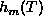(= metric entropy) is defined and finite. Pesin theory developed in  for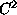-diffeomorphisms and generalized by Katok and Strelcyn  to systems with singularities, states that positive metric entropy is equivalent with the fact that an iterate of the map T is measure theoretically conjugated to a Bernoulli shift on a set of positive measure. In the case of a two-dimensional manifold X like in the billiard case, the Ruelle-Pesin-formulareduces the calculation of the metric entropyto the determination of the averaged Lyapunov exponent. This formula is also true for maps with singularities satisfying the Katok-Strelcyn conditions in [11, 13]. The billiard maps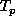studied here satisfy this condition.

Let us mention here also that the entropyof a monotone twist map is related to a determinant of an associated discrete Schrödinger operator L which is the Hessian of a natural variational problem associated to the dynamics. By the Thouless formula, one has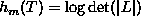(see ).

Positive Lyapunov exponents are by Wojtkowsky's theorem equivalent to the existence of an invariant cone bundle in the projective tangent bundle. For billiards, a formula of Wojtkowsky  gives a geometrical sufficient condition for the existence of an invariant cone bundle or using quadratic forms . For a refinement of these methods see for example . Unfortunately, no other mathematical tool is yet available to prove mathematically positive Lyapunov exponents for billiards. We computed them numerically.

In the case of the billiard map, there are coordinates (see ) in which the Jacobian of the billiard map can be put into the form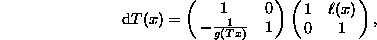where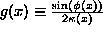. Hereis the curvature at an impact point,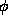is the angle of impact and l is the length of the trajectory between two successive impact points. In our numerical calculations, we took this as the Jacobian.

The first graph Fig 3. shows the entropy of the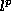-billiard for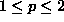. The Kolmogorov entropy was measured for 200 values of p between 1 and 2. For each p the Kolmogorov entropy was found by calculating the Lyapunov exponent at each point of agrid of initial conditions. At each of these 289 points the Lyapunov exponent was estimated as the value of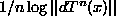for n=25,000 and the average over the 289 initial conditions was plotted.The next graph Fig. 4. shows the measured entropy of the-billiard for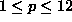(larger values of p introduce unreasonable numerical errors). We computed the Kolmogorov entropy for 200 values of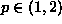and 1000 values of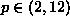. We again measured each Kolmogorov entropy by determining the average Lyapunov exponent over a 17 x 17 grid of initial conditions. The length of each orbit was n=3,461.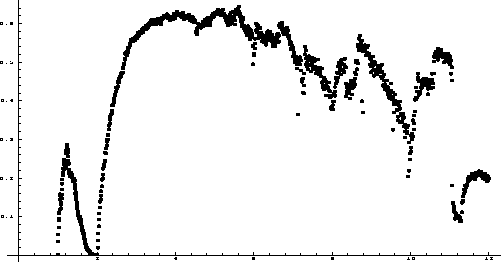As expected, the Kolmogorov entropy tends towards 0 for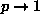and for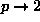. For, we measured a maximum entropy of 0.28 at p=1.23. For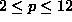, the entropy peaked around p=4 to p=6 with an entropy around 0.65.

Comparison between measurements of the entropy for orbits of length 3,461 and orbits of length 25,000 gave differences smaller than 0.05. The graphs indicate, that the entropy is piecewise monotone but that there are bumps.

We look now at the behavior of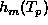near p=2. The measurements show thatwith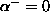and. We observe so a phase transition at p=2. The entropy grows with different growth rates for p;SPMgt;2 and p;SPMlt;2.

We show the numerical values of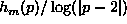in Fig. 5. We computed the entropy for 400 different values of p between 1.8 and 2.2 using again a 17 x 17 grid of initial points with each 5000 iterations so that for this run, 578 million billiard iterations had to be computed and for each orbit, the Jacobianevaluated.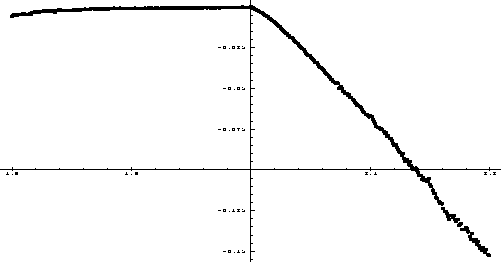Next: Discussion and open questions Up: Billiards in the unit Previous: Invariant curves and topological

Oliver Knill, Jul 10 1998